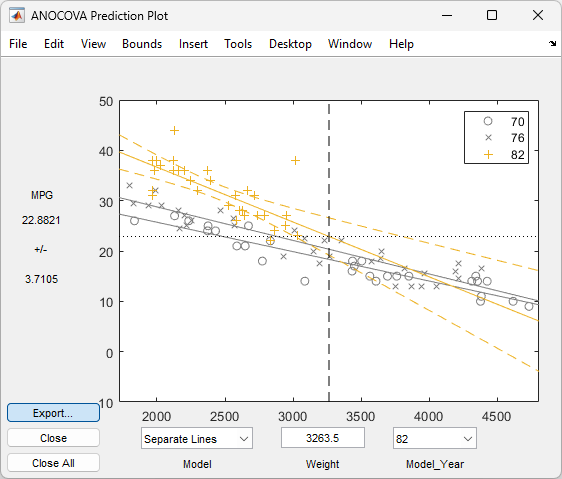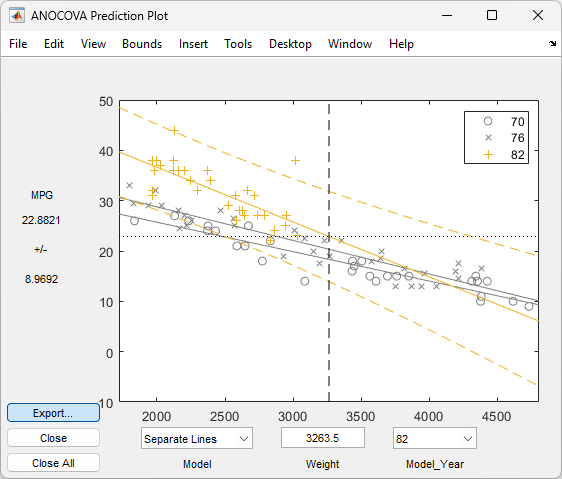## Analysis of Covariance

### Introduction to Analysis of Covariance

Analysis of covariance is a technique for analyzing grouped data having a response (y, the variable to be predicted) and a predictor (x, the variable used to do the prediction). Using analysis of covariance, you can model y as a linear function of x, with the coefficients of the line possibly varying from group to group.

### Analysis of Covariance Tool

The `aoctool` function opens an interactive graphical environment for fitting and prediction with analysis of covariance (ANOCOVA) models. It fits the following models for the ith group:

 Same mean y = α + ε Separate means y = (α + αi) + ε Same line y = α + βx + ε Parallel lines y = (α + αi) + βx + ε Separate lines y = (α + αi) + (β + βi)x + ε

For example, in the parallel lines model the intercept varies from one group to the next, but the slope is the same for each group. In the same mean model, there is a common intercept and no slope. In order to make the group coefficients well determined, the tool imposes the constraints

`$\sum {\alpha }_{j}=\sum {\beta }_{j}=0$`

The following steps describe the use of `aoctool`.

1. Load the data. The Statistics and Machine Learning Toolbox™ data set `carsmall.mat` contains information on cars from the years 1970, 1976, and 1982. This example studies the relationship between the weight of a car and its mileage, and whether this relationship has changed over the years. To start the demonstration, load the data set.

`load carsmall`

The Workspace Browser shows the variables in the data set.You can also use `aoctool` with your own data.

2. Start the tool. The following command calls `aoctool` to fit a separate line to the column vectors `Weight` and `MPG` for each of the three model group defined in `Model_Year`. The initial fit models the y variable, `MPG`, as a linear function of the x variable, `Weight`.

```[h,atab,ctab,stats] = aoctool(Weight,MPG,Model_Year); ```

See the `aoctool` function reference page for detailed information about calling `aoctool`.

3. Examine the output. The graphical output consists of a main window with a plot, a table of coefficient estimates, and an analysis of variance table. In the plot, each `Model_Year` group has a separate line. The data points for each group are coded with the same color and symbol, and the fit for each group has the same color as the data points.The coefficients of the three lines appear in the figure titled ANOCOVA Coefficients. You can see that the slopes are roughly –0.0078, with a small deviation for each group:

• Model year 1970: y = (45.9798 – 8.5805) + (–0.0078 + 0.002)x + ε

• Model year 1976: y = (45.9798 – 3.8902) + (–0.0078 + 0.0011)x + ε

• Model year 1982: y = (45.9798 + 12.4707) + (–0.0078 – 0.0031)x + εBecause the three fitted lines have slopes that are roughly similar, you may wonder if they really are the same. The `Model_Year*Weight` interaction expresses the difference in slopes, and the ANOVA table shows a test for the significance of this term. With an F statistic of 5.23 and a p value of 0.0072, the slopes are significantly different.4. Constrain the slopes to be the same. To examine the fits when the slopes are constrained to be the same, return to the ANOCOVA Prediction Plot window and use the Model pop-up menu to select a `Parallel Lines` model. The window updates to show the following graph.Though this fit looks reasonable, it is significantly worse than the `Separate Lines` model. Use the Model pop-up menu again to return to the original model.

### Confidence Bounds

The example in Analysis of Covariance Tool provides estimates of the relationship between `MPG` and `Weight` for each `Model_Year`, but how accurate are these estimates? To find out, you can superimpose confidence bounds on the fits by examining them one group at a time.

1. In the Model_Year menu at the lower right of the figure, change the setting from `All Groups` to 82. The data and fits for the other groups are dimmed, and confidence bounds appear around the 82 fit.The dashed lines form an envelope around the fitted line for model year 82. Under the assumption that the true relationship is linear, these bounds provide a 95% confidence region for the true line. Note that the fits for the other model years are well outside these confidence bounds for `Weight` values between `2000` and `3000`.

2. Sometimes it is more valuable to be able to predict the response value for a new observation, not just estimate the average response value. Use the `aoctool` function Bounds menu to change the definition of the confidence bounds from `Line` to `Observation`. The resulting wider intervals reflect the uncertainty in the parameter estimates as well as the randomness of a new observation.Like the `polytool` function, the `aoctool` function has cross hairs that you can use to manipulate the `Weight` and watch the estimate and confidence bounds along the y-axis update. These values appear only when a single group is selected, not when ```All Groups``` is selected.

### Multiple Comparisons

You can perform a multiple comparison test by using the `stats` output structure from `aoctool` as input to the `multcompare` function. The `multcompare` function can test either slopes, intercepts, or population marginal means (the predicted MPG of the mean weight for each group). The example in Analysis of Covariance Tool shows that the slopes are not all the same, but could it be that two are the same and only the other one is different? You can test that hypothesis.

```multcompare(stats,0.05,'on','','s') ans = 1.0000 2.0000 -0.0012 0.0008 0.0029 1.0000 3.0000 0.0013 0.0051 0.0088 2.0000 3.0000 0.0005 0.0042 0.0079 ```

This matrix shows that the estimated difference between the intercepts of groups 1 and 2 (1970 and 1976) is 0.0008, and a confidence interval for the difference is [–0.0012, 0.0029]. There is no significant difference between the two. There are significant differences, however, between the intercept for 1982 and each of the other two. The graph shows the same information.Note that the `stats` structure was created in the initial call to the `aoctool` function, so it is based on the initial model fit (typically a separate-lines model). If you change the model interactively and want to base your multiple comparisons on the new model, you need to run `aoctool` again to get another `stats` structure, this time specifying your new model as the initial model.